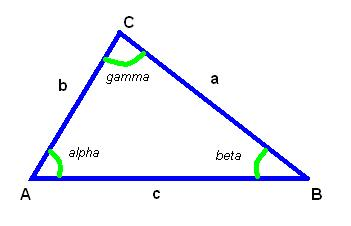homechevron_rightStudychevron_rightMathchevron_rightGeometry

# Lengths of triangle sides given one side and two angles

This online calculator determines lengths of triangle sides given one side and two angles

### This page exists due to the efforts of the following people:

#### TimurWell, there are myriad different ways to do math with a triangle. I guess it is because a triangle is a fundamental shape in geometry. This calculator is for those who wanted to determine lengths of triangle sides given one side and two angles.

It is actually simple, you just need to use law of sines, which looks like this:

That's it.#### Lengths of triangle sides given one side and two angles

°
°
Digits after the decimal point: 2
Side A

Side B

URL copied to clipboard

#### Similar calculatorsPLANETCALC, Lengths of triangle sides given one side and two angles